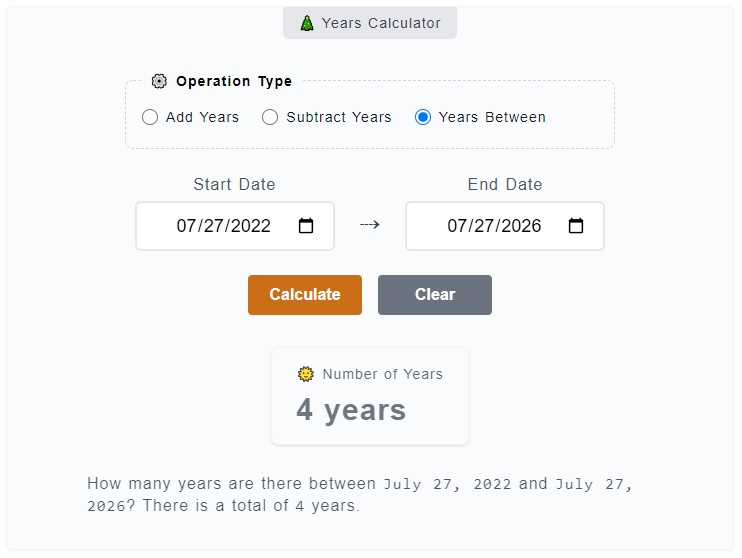# Years Calculator

The Years Calculator has three (3) operations, it can get the number of years between two dates, add years and subtract years from a starting date. For example, you can add `11 years` or subtract `5` years from the starting date. You can also get the years between and . Just enter these values on the calculator.

🎄 Years Calculator
⚙️ Operation Type

### 🌞 Number of Years

4 years

How many years are there between `June 23, 2023` and `June 23, 2027`? There is a total of `4` years.

## How to Use the Years Calculator

To use the Years Calculator, you just need to pick first the operation you want to use - add years, subtract years or get the total number of years in between two dates. You just need to enter the values on corresponding inputs and click on `Calculate` button. Here's a more detailed step by step guide.1. Step 1

On the topmost part of the calculator, you can find the `⚙️ Operation Type` field set. It is where you need to choose from the operation options - add years, subtract years and years between. For this example, let's select the `years between` option which is already selected by default.

2. Step 2

After you have chosen an operation type, the next thing to do is to fill the input fields - `Start Date` and `End Date`. The Start Date represents the base or reference date of the count-off while the End Date is where the count-off ends.

3. Step 3

The final step is to click on the `Calculate` button to get your results. You can reset the filled input fields as well by just clicking on the `Clear` button.

In case you are doing some addition or subtraction of years from the starting date, first select the `add` or `subtract` as the type of operation. Then, enter the starting date and the number of days you want to add or subtract on their corresponding fields.

Although we have a much specific calculator for years from now (➕ add years) and years ago (➖ subtract years), this calculator has more option to change the starting date and combined with years between two dates operation.